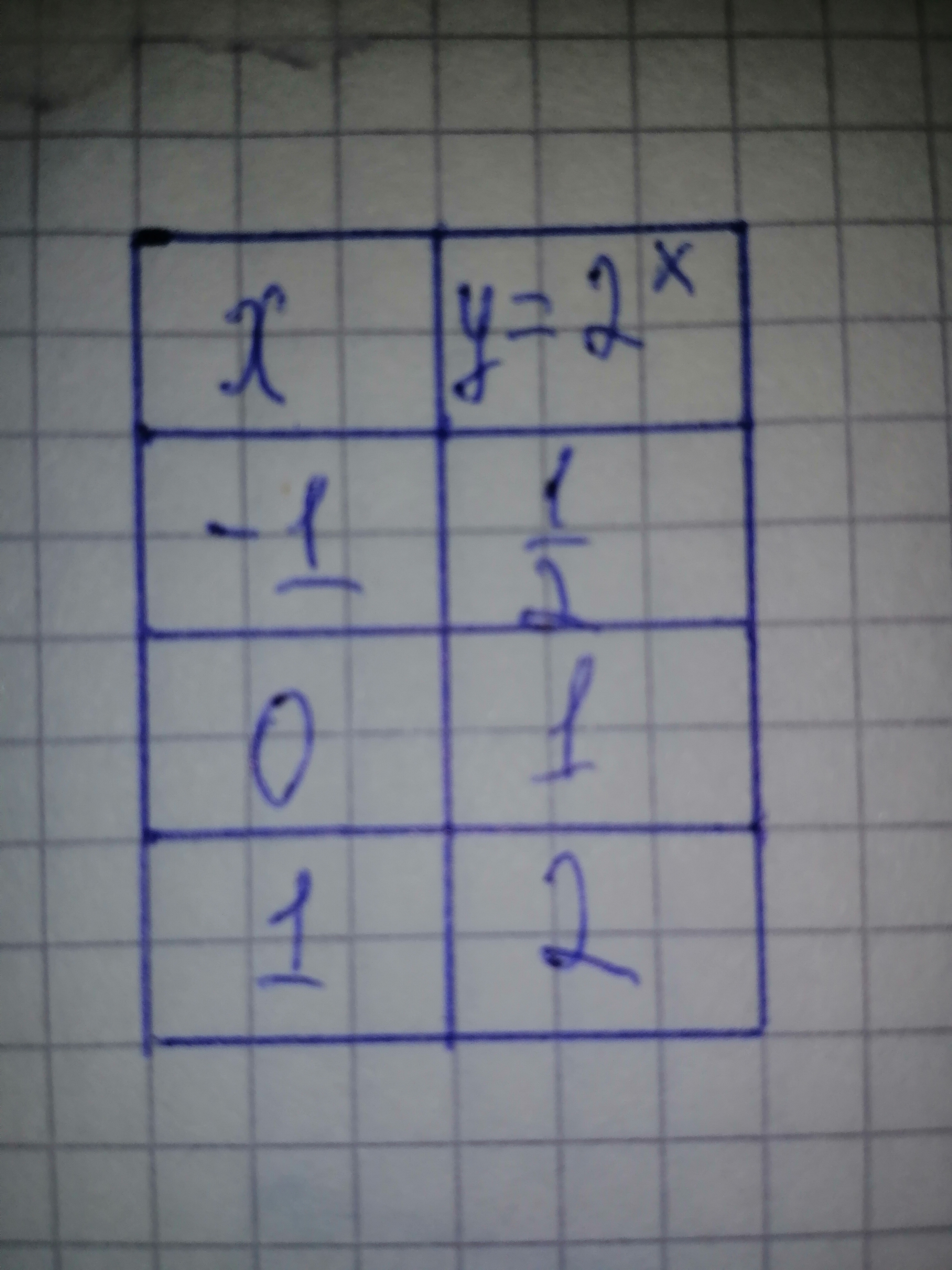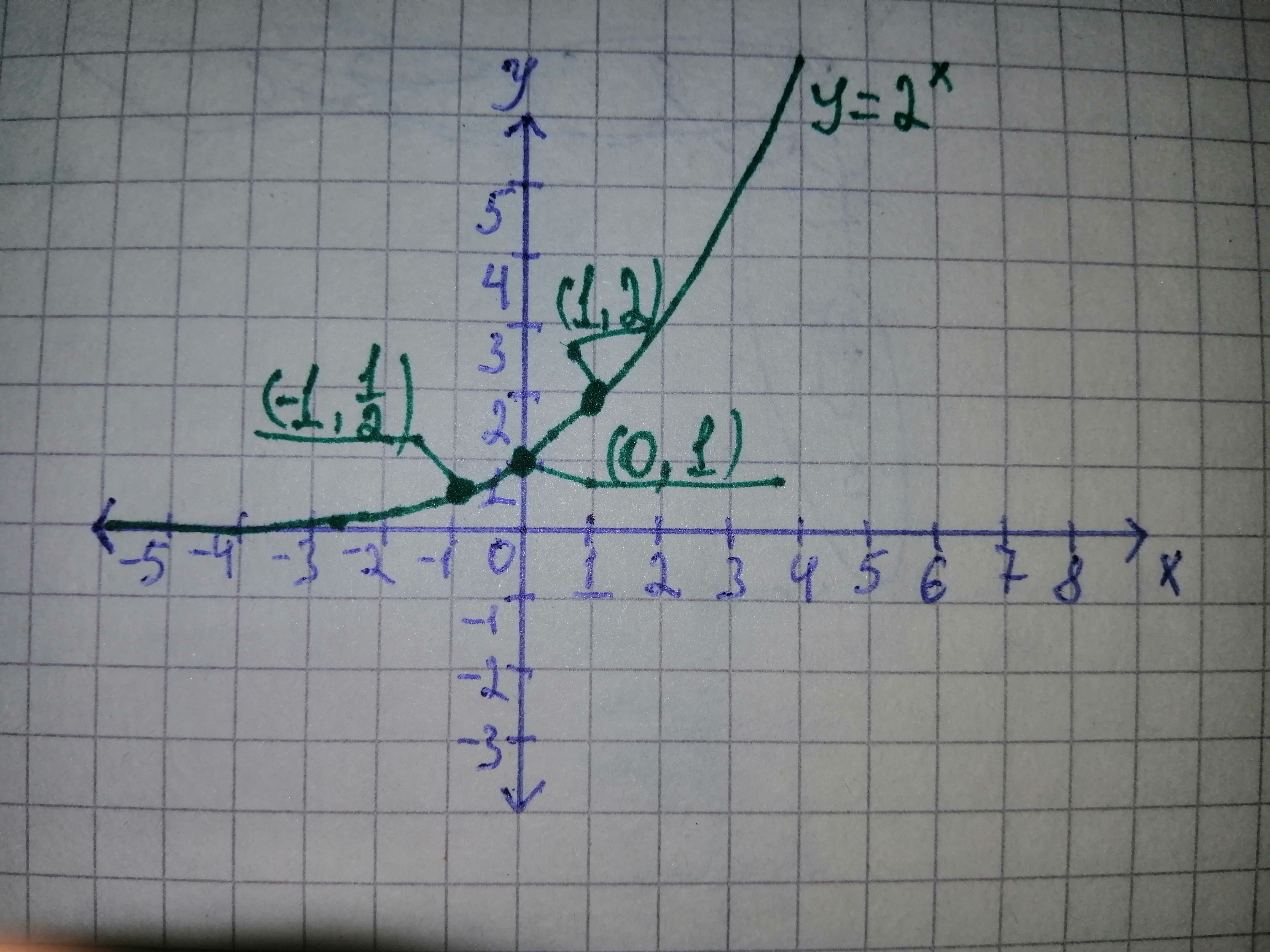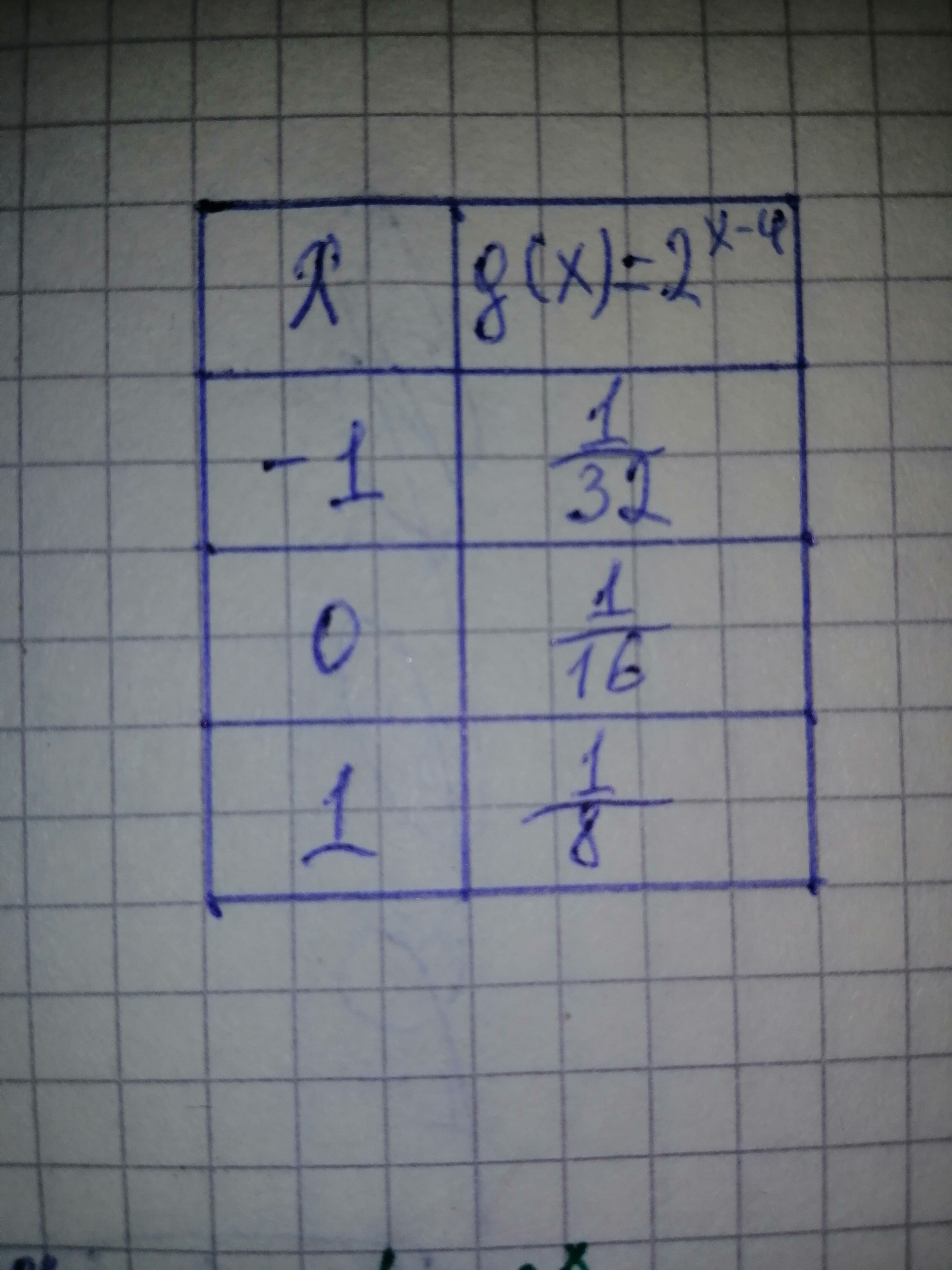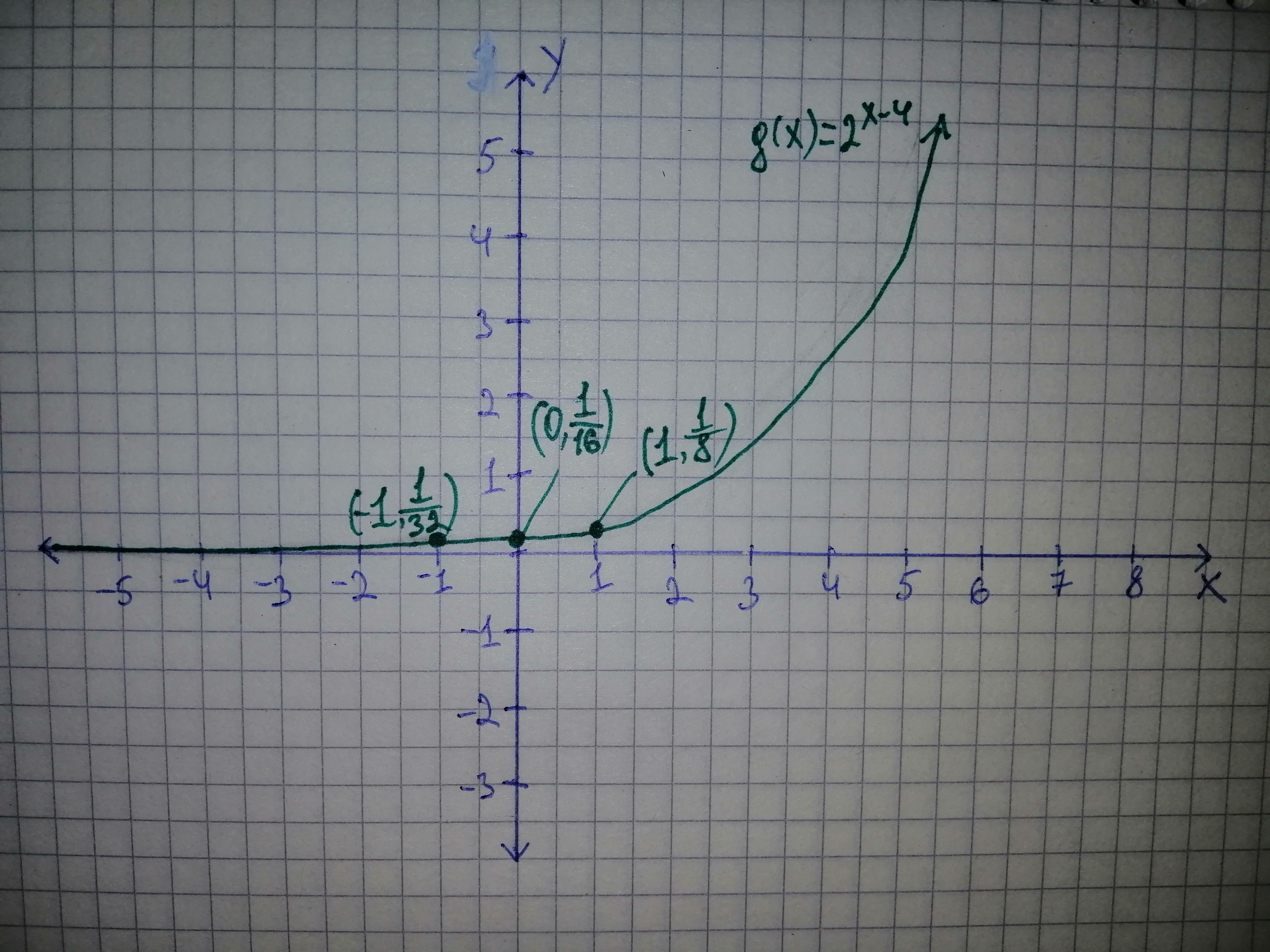# Complete the tasks to determine: a)To graf: The function g{{left({x}right)}}={2}^{{{x}-{4}}}. b)The domain of the function g{{left({x}right)}}={2}^{{{x}-{4}}} in the interval notation. c)The range of the function g{{left({x}right)}}={2}^{{{x}-{4}}} in the interval notation. d)The equation of the asymptote of the function g{{left({x}right)}}={2}^{{{x}-{4}}}.Question
Transformation propertiesa)To graf: The function $$g{{\left({x}\right)}}={2}^{{{x}-{4}}}.$$
b)The domain of the function $$g{{\left({x}\right)}}={2}^{{{x}-{4}}}$$ in the interval notation.
c)The range of the function $$g{{\left({x}\right)}}={2}^{{{x}-{4}}}$$ in the interval notation.
d)The equation of the asymptote of the function $$g{{\left({x}\right)}}={2}^{{{x}-{4}}}.$$2021-03-13
a) Graph:
Use the transformation of the graph of the parent function $${y}={2}^{x}$$ to
graph the given function $$g{{\left({x}\right)}}={2}^{{{x}-{4}}}.$$
First, draw the graph of $${y}={2}^{x}.$$ For this purpose, draw the table for the
different points on the graph of the parent function $${y}={2}^{x}.$$
Substitute - 1 for x in the above parent function $${y}={2}^{x}.$$
$${y}={2}^{ -{{1}}}$$
$$= \frac{1}{{2}}$$
Now, substitute 0 for x .
$${y}={2}^{0}$$
$$= 1$$
Finally, substitute 1 for x.
$${y}={2}^{1}$$
$$= 2$$
Thus, the table for the graph of the parent function $${y}={2}^{x}$$ is,Now, consider the original function $$g{{\left({x}\right)}}={2}^{{{x}-{4}}}.$$ Use the properties of the transformation of the graph. In the graph of the function
$$g{{\left({x}\right)}}={2}^{{{x}-{4}}},$$
the parent function graph $${y}={2}^{x}$$ is shifted to 4 units
positive towards the right.
Similarly, draw the table for the graph of the original function
$$g{{\left({x}\right)}}={2}^{{{x}-{4}}}$$Refer to the above table and draw the graph of the original function $$g{{\left({x}\right)}}={2}^{{{x}-{4}}}$$From the graph, it observed that function $$g{{\left({x}\right)}}={2}^{{{x}-{4}}}$$
is increasing in the domain $$(-\infty, \infty).$$
The graph of the function $$g{{\left({x}\right)}}={2}^{{{x}-{4}}}$$ shifts 4 units towards the right along the abscissa by using this property of transformation of graph.
b) The domain is the set of all the inputs for which the given function is defined and is real.
Refer to the graph of the given function $$g{{\left({x}\right)}}={2}^{{{x}-{4}}}$$ in subpart (a).
From the graph, it can be concluded that the given function
$$g{{\left({x}\right)}}={2}^{{{x}-{4}}}$$ is defined for all the real values of x.
Thus, write the obtained domain in interval notation. $$(- \infty, \infty)$$
Therefore, the domain of the given function is (- \infty, \infty).
c)
The range of the given function is the set of all the dependent variables for which it is defined.
Refer to the graph of the given function $$g{{\left({x}\right)}}={2}^{{{x}-{4}}}$$ in subpart (a).
From the graph, it can be concluded that the given function
$$g{{\left({x}\right)}}={2}^{{{x}-{4}}}$$ gives the output value above the y-axis.
Thus, write the obtained range in the interval notation.
$$(0, \infty)$$
Therefore, the range of the given function is $$(0, pp).$$
(d)
An asymptote of a curve is a line such that the distance between the curve and the line approaches zero as one or both of the x or y coordinates tend to infinity.
Refer to the graph of the function $$g{{\left({x}\right)}}={2}^{{{x}-{4}}}$$ in subpart (a).
From the graph, it can be concluded that the equation for the asymptote of the given function $$g{{\left({x}\right)}}={2}^{{{x}-{4}}}{i}{s}{y}={0}.$$
Therefore, the equation for the asymptote of the given function is
$$y = 0$$.

### Relevant Questionsa) Find the parent function f.
Given Information: $$g{{\left({x}\right)}}=-{2}{\left|{x}-{1}\right|}-{4}$$
b) Find the sequence of transformation from f to g.
Given information: $$f{{\left({x}\right)}}={\left[{x}\right]}$$
c) To sketch the graph of g.
Given information: $$g{{\left({x}\right)}}=-{2}{\left|{x}-{1}\right|}-{4}$$
d) To write g in terms of f.
Given information: $$g{{\left({x}\right)}}=-{2}{\left|{x}-{1}\right|}-{4}{\quad\text{and}\quad} f{{\left({x}\right)}}={\left[{x}\right]}$$For $$\displaystyle{y}=\ -{{\log}_{{{2}}}{x}}$$.
a) Use transformations of the graphs of $$\displaystyle{y}={{\log}_{{{2}}}{x}}$$ and $$\displaystyle{y}={{\log}_{{{3}}}{x}}$$ o graph the given functions.
b) Write the domain and range in interval notation.
c) Write an equation of the asymptote.Use function notation to describe the way the second variable (DV) depends upon the first variable (IV). Determine the domain and range for each, determine if there is a positive, negative, or no relationship, and explain your answers.
A)IV: an acute angle V in a right triangle: DV: the area B of the triangle if the hypotenuse is a fixed length G.
B)IV: one leg P of a right triangle: DV: the hypotenuse G of the right triangle if the other leg is 2
C)IV: the hypotenuse G of a right triangle: DV: the other leg P of the right triangle is one leg is 5.a) Find the sequence of transformation from f to g
Given information: $$g{{\left({x}\right)}}=\frac{1}{{2}}{\left|{x}-{2}\right|}-{3}{\quad\text{and}\quad} f{{\left({x}\right)}}={x}^{3}$$
b) To sketch the graph of g.
Given information: $$f{{\left({x}\right)}}={\left|{x}\right|}$$
c) To write g in terms of f.
Given information: $$g{{\left({x}\right)}}=\frac{1}{{2}}{\left|{x}-{2}\right|}-{3}{\quad\text{and}\quad} f{{\left({x}\right)}}={\left|{x}\right|}$$Aurora is planning to participate in an event at her school's field day that requires her to complete tasks at various stations in the fastest time possible. To prepare for the event, she is practicing and keeping track of her time to complete each station. The x-coordinate is the station number, and the y-coordinate is the time in minutes since the start of the race that she completed the task. $$\displaystyle{\left({1},{3}\right)},{\left({2},{6}\right)},{\left({3},{12}\right)},{\left({4},{24}\right)}$$
Part A: Is this data modeling an algebraic sequence or a geometric sequence? Explain your answer.
Part B: Use a recursive formula to determine the time she will complete station 5.
Part C: Use an explicit formula to find the time she will complete the 9th station.The table shows some values of the derivative of an unknown function f.Complete the table by finding the derivative of each transformation of f, it possible
a) $$g(x) = f(x)\ -\ 2$$
b) $$h(x) = 2 f(x)$$
c) $$r(x) = f(-3x)$$a) To graph: the $${k}{e}{r}{\left({A}\right)},{\left({k}{e}{r}{A}\right)}^{\bot}{\quad\text{and}\quad}{i}{m}{\left({A}^{T}\right)}$$
b) To find: the relationship between im $$(A^{T})$$ and ker (A).
c) To find: the relationship between ker(A) and solution set S
d) To find vecx_0 at the intersection of $${k}{e}{r}{\left({A}\right)}{\quad\text{and}\quad}{\left({k}{e}{r}{A}\right)}^{\bot}$$
e) To find: the lengths of $$\vec{{x}}_{{0}}$$ compared to the other vectors in Sa) Identify the parameters a, k, d, and c in the polynomial function $$\displaystyle{y}={\frac{{{1}}}{{{3}}}}{\left[-{2}{\left({x}+{3}\right)}\right]}^{{{4}}}-{1}$$. Describe how each parameter transforms the base function $$\displaystyle{y}={x}^{{{4}}}$$. b) State the domain and range, the vertex, and the equation of the axis of symmetry of the transformed function. c) Describe two possible orders in which the transformations can be applied to the graph of $$\displaystyle{y}={x}^{{{4}}}$$ to produce the graph of $$\displaystyle{y}={\frac{{{1}}}{{{3}}}}{\left[-{2}{\left({x}+{3}\right)}\right]}^{{{4}}}-{1}$$. d) Sketch graphs of the base function and the transformed function on the same set of axes.An automobile tire manufacturer collected the data in the table relating tire pressure x​ (in pounds per square​ inch) and mileage​ (in thousands of​ miles). A mathematical model for the data is given by
$$\displaystyle​ f{{\left({x}\right)}}=-{0.554}{x}^{2}+{35.5}{x}-{514}.$$
$$\begin{array}{|c|c|} \hline x & Mileage \\ \hline 28 & 45 \\ \hline 30 & 51\\ \hline 32 & 56\\ \hline 34 & 50\\ \hline 36 & 46\\ \hline \end{array}$$
​(A) Complete the table below.
$$\begin{array}{|c|c|} \hline x & Mileage & f(x) \\ \hline 28 & 45 \\ \hline 30 & 51\\ \hline 32 & 56\\ \hline 34 & 50\\ \hline 36 & 46\\ \hline \end{array}$$
​(Round to one decimal place as​ needed.)
$$A. 20602060xf(x)$$
A coordinate system has a horizontal x-axis labeled from 20 to 60 in increments of 2 and a vertical y-axis labeled from 20 to 60 in increments of 2. Data points are plotted at (28,45), (30,51), (32,56), (34,50), and (36,46). A parabola opens downward and passes through the points (28,45.7), (30,52.4), (32,54.7), (34,52.6), and (36,46.0). All points are approximate.
$$B. 20602060xf(x)$$
Acoordinate system has a horizontal x-axis labeled from 20 to 60 in increments of 2 and a vertical y-axis labeled from 20 to 60 in increments of 2.
Data points are plotted at (43,30), (45,36), (47,41), (49,35), and (51,31). A parabola opens downward and passes through the points (43,30.7), (45,37.4), (47,39.7), (49,37.6), and (51,31). All points are approximate.
$$C. 20602060xf(x)$$
A coordinate system has a horizontal x-axis labeled from 20 to 60 in increments of 2 and a vertical y-axis labeled from 20 to 60 in increments of 2. Data points are plotted at (43,45), (45,51), (47,56), (49,50), and (51,46). A parabola opens downward and passes through the points (43,45.7), (45,52.4), (47,54.7), (49,52.6), and (51,46.0). All points are approximate.
$$D.20602060xf(x)$$
A coordinate system has a horizontal x-axis labeled from 20 to 60 in increments of 2 and a vertical y-axis labeled from 20 to 60 in increments of 2. Data points are plotted at (28,30), (30,36), (32,41), (34,35), and (36,31). A parabola opens downward and passes through the points (28,30.7), (30,37.4), (32,39.7), (34,37.6), and (36,31). All points are approximate.
​(C) Use the modeling function​ f(x) to estimate the mileage for a tire pressure of 29
$$\displaystyle​\frac{{{l}{b}{s}}}{{{s}{q}}}\in.$$ and for 35
$$\displaystyle​\frac{{{l}{b}{s}}}{{{s}{q}}}\in.$$
The mileage for the tire pressure $$\displaystyle{29}\frac{{{l}{b}{s}}}{{{s}{q}}}\in.$$ is
The mileage for the tire pressure $$\displaystyle{35}\frac{{{l}{b}{s}}}{{{s}{q}}}$$ in. is
(Round to two decimal places as​ needed.)
(D) Write a brief description of the relationship between tire pressure and mileage.
A. As tire pressure​ increases, mileage decreases to a minimum at a certain tire​ pressure, then begins to increase.
B. As tire pressure​ increases, mileage decreases.
C. As tire pressure​ increases, mileage increases to a maximum at a certain tire​ pressure, then begins to decrease.
D. As tire pressure​ increases, mileage increases.$${y}{''}+{4}{y}= \sin{{t}}+{u}_{\pi}{\left({t}\right)} \sin{{\left({t}-\pi\right)}}:$$
$$y(0) = 0,$$
$$y'(0) = 0.$$